# Test: Evaluating Definite Integrals

## 10 Questions MCQ Test Mathematics (Maths) Class 12 | Test: Evaluating Definite Integrals

Description
This mock test of Test: Evaluating Definite Integrals for JEE helps you for every JEE entrance exam. This contains 10 Multiple Choice Questions for JEE Test: Evaluating Definite Integrals (mcq) to study with solutions a complete question bank. The solved questions answers in this Test: Evaluating Definite Integrals quiz give you a good mix of easy questions and tough questions. JEE students definitely take this Test: Evaluating Definite Integrals exercise for a better result in the exam. You can find other Test: Evaluating Definite Integrals extra questions, long questions & short questions for JEE on EduRev as well by searching above.
QUESTION: 1

### Evaluate: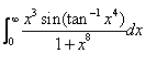Solution:

Let I = ∫(0 to pi/2)x3 sin(tan−1x4)/(1+x8)dx
Put tan−1 x4 = t
4x3/(1+x8)dx = dt
⇒x3dx/(1+x8) = dt/4
Now, I = 1/4∫sin t dt
=−[1/4 cos t]0 to pi/2 + c
= -1/4[cos(0) - cos(pi/2)] +c
= 1/4[-cos(pi/2) + cos(0)] +c
= ¼[-0+1]
= 1/4

QUESTION: 2

###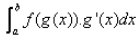Solution:

The given form of integral function (say ∫f(x)) can be transformed into another by changing the independent variable x to t,
Substituting x = g(t) in the function ∫f(x), we get;
dx/dt = g'(t)
or dx = g'(t).dt
Thus, I = ∫f(x).dx = f(g(t)).g'(t).dt
Therefore,  ∫f(g(x)).g'(x).dx  = f(b) - f(a)

QUESTION: 3

###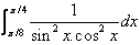Solution:

∫(π/8 to π/4)1/(sin2x cos2x)
= ∫(π/8 to π/4)(sin2x + cos^2x)/(sin2x cos2x) dx
= ∫(π/8 to π/4)(1/cos2x +1/sin2x) dx
= ∫(π/8 to π/4)(sec2 x dx + cosec2 x dx)
= [tan x - cot x](π/8 to π/4) + c
= [tan(π/8) - tan(π/4)] - [cot((π/8) - cot(π/4)]
= ((2)½ +1  - 1) - (1/(2)½ +1 - 1)
= 2

QUESTION: 4

The value of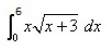is:

Solution:
QUESTION: 5

If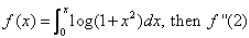is

Solution:

In the question, it should be f’(2) instead of f”(2)
Explanation:- f(x) = ∫(0 to x) log(1+x2)
f’(x) = 2xdx/(1+x2)
f’(2) = 2(2)/(1+(2)2)
= 4/5

QUESTION: 6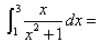Solution:

∫(1 to 3) x/(1+x2) dx
Put t = 1+x2
dt = 2x dx
= 1/2∫( 1 to 3) dt/t
= ½  log[t](1 to 3)
= ½[log(1+x2)](1 to 3)
= ½[log 10 - log 2]
= ½ (log(10/2)]
= ½[log 5]
= log(5)½

QUESTION: 7

If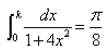then the value of k is:

Solution:

Let I=∫(0 to k) 1/[1 + 4x2]dx = π8
Now, ∫(0 to k) 1/[4(1/4 + x2)]dx
= 2/4[tan−1 2x]0 to k
= 1/2tan-1 2k − 0 = π/8
1/2tan−1 2k = π8
⇒ tan−1 2k = π/4
⇒ 2k = 1
∴ k = 1/2

QUESTION: 8

Evaluate: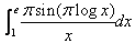Solution:
QUESTION: 9

The value of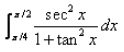is:​

Solution:

1 + tan2 x = sec2 x
∫(π/2 to π/4)(sec2 x)/(1 + tan2 x)
=> ∫(π/2 to π/4)(sec2 x)/(sec2 x) dx
= ∫1(π/2 to π/4) dx
= [x](π/2 to π/4)
= π/2 - π/4  =  π/4

QUESTION: 10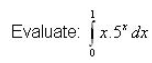Solution:

∫x(5x) dx
u = x,   dv = 5x dx
du = dx,   v = (5x/ln 5)
∫x(5x) dx = [x(5x)/ln 5](0 to 1) - ∫(0 to 1)(5x)/ln 5 dx
= 5/ ln 5 - 0 - 1/ln 5[5x/ln 5](0 to 1)
= 5/ln 5 - 5/(ln 5)2 + 1/(ln 5)2
=5/ln 5 - 4/(ln 5)2